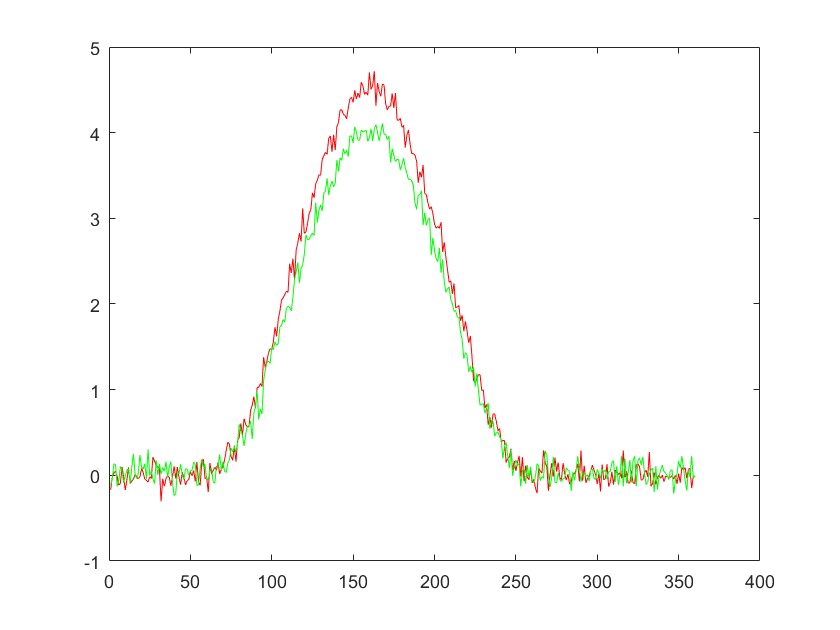init_unfold
Starting unfold toolbox. Adding subfolders and other toolboxes to path... Done.

# Introduction

This basic tutorial starts with generating some EEG data with overlap. For the sake of having a backstory, we will simulate data of an experiment where we have two types of stimuli (face or car) with two types of colors (red and green) embedded in pink noise. Subjects are asked to count the number of objects irregardless of color.
We are interested what the influences of the stimulus-type and color is.
In this tutorial we will do the following:
• generate and plot the designmatrix
• timeexpand the designmatrix and visualize it
% We will first simulate data based on the car/face, green/red design.
EEG = tutorial_simulate_data('2x2');
eeg_checkset note: upper time limit (xmax) adjusted so (xmax-xmin)*srate+1 = number of frames
The necessary fields of the EEG-structure are:
fprintf('the continuous data EEG.data: %i channels and %i samples\n',size(EEG.data))
the continuous data EEG.data: 1 channels and 120000 samples
fprintf('the sampling rate EEG.srate: %i \n',EEG.srate)
the sampling rate EEG.srate: 200
fprintf('a structure of events EEG.event: %i \n',length(EEG.event))
a structure of events EEG.event: 413
The EEG.event structure is defined by eeglab with the following fields:
• EEG.event.latency % the latency in samples (can be uneven)
• EEG.event.type % the type of the event
• EEG.event.customField1 % custom fields1 (i.e. stimulusType)
Once you specified these fields in a structure you are ready to go!
Let's see what fields we have simulated:
EEG.event(1)
ans = struct with fields:
latency: 92 type: 'fixation' intercept: 1 stimulusType: 'face' color: 'red'

# Defining the 2x2 factorial design

A 2x2 anova can be reduced to a linear model with two categorical predictors and the interaction. We adopt the widely popular 'formula'-style of model definition. In this case 'y ~ A + B + A:B' (equivalent: 'y ~ A*B') represents the whole model. A and B are our main effects, A:B the interaction. We should specify that we have two categorical factors and we have to specify on which event(s) we want to epoch our data and evaluate the model.
cfgDesign = [];
cfgDesign.eventtypes = {'fixation'};
cfgDesign.formula = 'y ~ 1+ cat(stimulusType)*cat(color)'
cfgDesign = struct with fields:
eventtypes: {'fixation'} formula: 'y ~ 1+ cat(stimulusType)*cat(color)'
EEG = uf_designmat(EEG,cfgDesign);
Modeling 413 event(s) of [fixation] using formula: y~1+stimulusType*color
subplot(2,1,1),uf_plotDesignmat(EEG,'sort',0,'figure',0)
subplot(2,1,2),uf_plotDesignmat(EEG,'sort',1,'figure',0)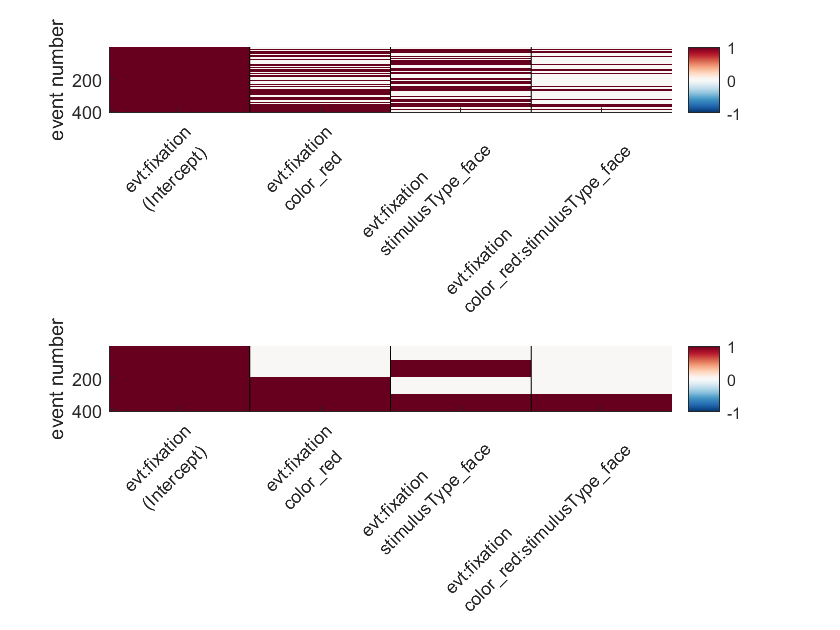The two plots show the designmatrix. On the top we have it unsorted, where the y-axis depicts the event in order of appearance. The bottom one is a sorted version. We will discuss the bottom plot. Each column represents a single predictor or effect. The first column has only ones. It is called the reference, the constant or the intercept. We are using treatment/reference coding (instead of effect-coding) and this assigns a special meaning to the first column: It represents the value of the ERP that is assigned, if all other predictors are zero. So with the second column indicating "color=red", the third "stimulusType=face" and the fourth the interaction, this means that the first column represents what a trial looks like when a green car is shown. The other predictors depict the respective difference-effect to this reference (what you have to add in order to go to another factor level).
A formula and some examples might make this more clear: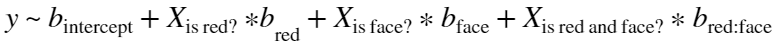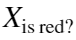can be instantiated with 0 or 1. Thus if we want to know a red car we would calculate: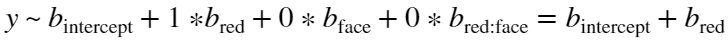For a red face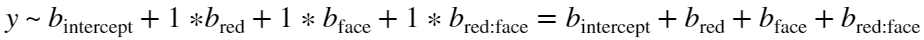And so on.

# Timeshift

In order to compensate for the inevitable linear overlap between ERPs, we want to use timeshifting / prepare the designmatrix for the deconvolution step. In this step we generate for each column of the designmatrix timeshifted (by t = 1, t=2, t=3 ...) and append them to the designmatrix.
cfgTimeshift = [];
cfgTimeshift.timelimits = [-0.3,1.5];
EEG = uf_timeexpandDesignmat(EEG,cfgTimeshift);
uf_timeexpandDesignmat(): Timeexpanding the designmatrix... ...done
uf_plotDesignmat(EEG,'timeexpand',1)
ylim([300 305])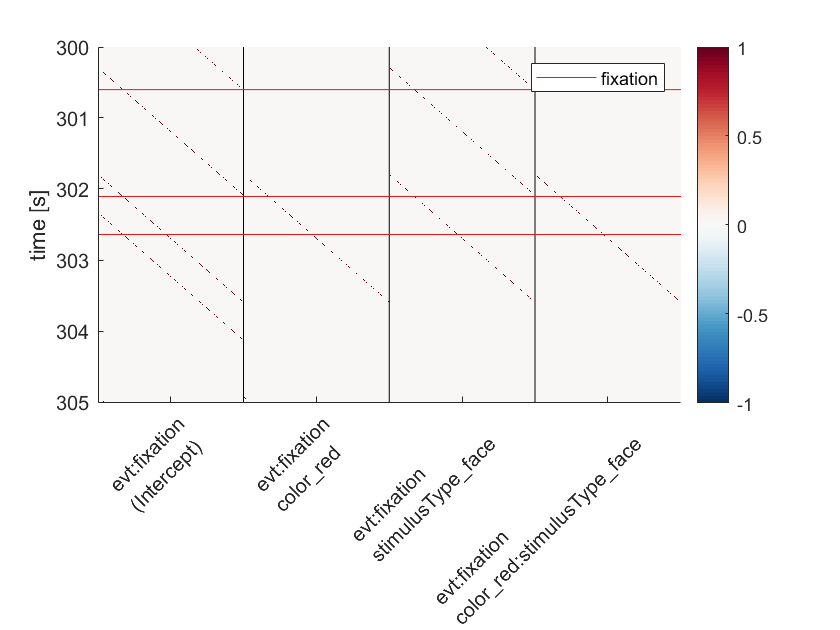This plot shows how the designmatrix works that achieves linear deconvolution. Each parameter in the designmatrix X spans now over the whole contious timeseries (n samples) instead of a single value per trial. This is done by an expansion of each column into a time-resolved set of columns. The new columns represent the predictor at a given time-point. If graphically we imagine that we predict some EEG-signal along the y/time-axis, then basically we try to predict one sample based on a horizontal slice through the matrix, weighted by to-be-estimated beta-parameters.

# Fit the model

We solve the linear equation: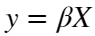(y=data, X= Designmatrix) for β at each point in time. These betas reflect our parameter estimates.
EEG= uf_glmfit(EEG); % this method is fast but needs lots of ram
uf_glmfit(): Fitting deconvolution model...solving the equation system solving electrode 1 (of 1 electrodes in total)... 35 iterations, took 0.1s LMfit finished

# Plot the results

We choose a single electrode and plot all effects.
ufresult = uf_condense(EEG);
cfg = [];
cfg.channel = 1;
ax = uf_plotParam(ufresult,cfg);
plotting all parameters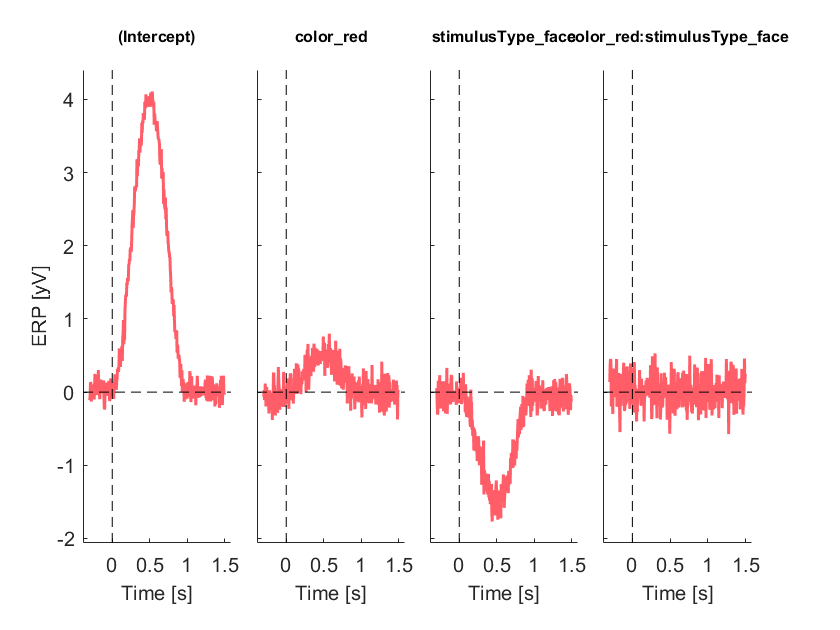One can clearly see that we recover the intercept and the simulated effects. The intercept represents the response when both stimulusType = 'car' and color = 'green'. The second column is the component one needs to add to the intercept to gain the ERP for the color 'red'. The third column equally represents the difference (i.e. what to add) of face to car. The fourth column represents the interaction. Because we did not simulate an interaction, this ERP is flat.
Sometimes it helps interpretation to add the intercept to the factors.
cfg.add_intercept = 1; % sum the intercept to each column
cfg.include_intercept = 1; % include the intercept as dashed line
ax = uf_plotParam(ufresult,cfg);
plotting all parameters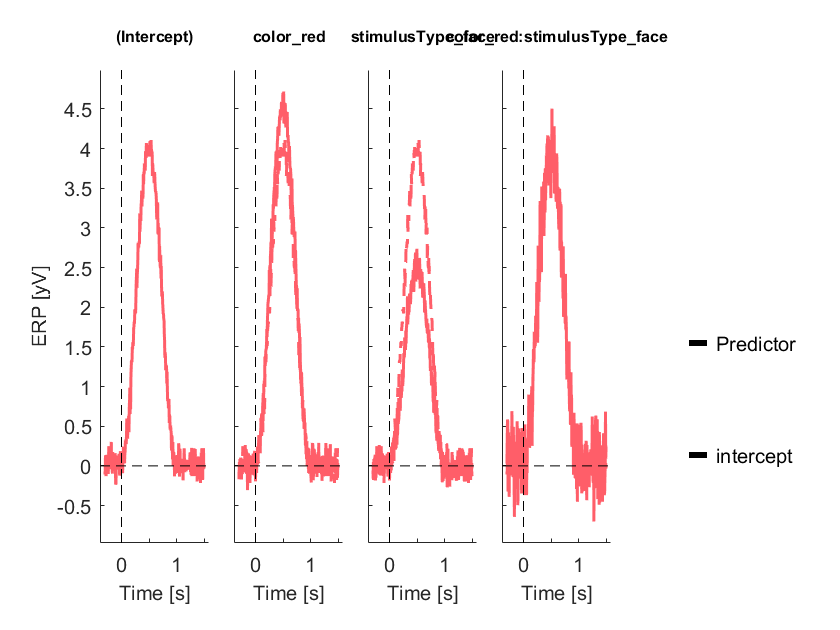Important: The interaction should NOT be interpreted with add_interception.

# The ufresult structure

We can have a look at ufresult:
ufresult
ufresult = struct with fields:
unfold: [1×1 struct] beta: [1×360×4 double] times: [1×360 double] chanlocs: [] param: [1×4 struct]
Here the ufresult.beta contains the beta for each time-point and the epochs represent different predictors at different evaluated levels (e.g. a continous predictor evaluated at the quartiles).
Information on which parameter corresponds to which predictors can be found in EEG.param
ufresult.param(2)
ans = struct with fields:
value: NaN name: 'color_red' event: {'fixation'} type: 'categorical'

# Treatment vs. Effect coding

So far we used treatment coding. There is also another popular coding scheme, effects coding.
cfgDesign.codingschema = 'effects'
cfgDesign = struct with fields:
eventtypes: {'fixation'} formula: 'y ~ 1+ cat(stimulusType)*cat(color)' codingschema: 'effects'
EEG = uf_designmat(EEG,cfgDesign);
Modeling 413 event(s) of [fixation] using formula: y~1+stimulusType*color
EEG = uf_timeexpandDesignmat(EEG,cfgTimeshift);
uf_timeexpandDesignmat(): Timeexpanding the designmatrix... ...done
EEG= uf_glmfit(EEG);
uf_glmfit(): Fitting deconvolution model...solving the equation system solving electrode 1 (of 1 electrodes in total)... 15 iterations, took 0.0s LMfit finished
% combine the two datasets
ufresult2 = uf_condense(EEG);
ufresult.effects = ufresult2.beta * 2; % * 2 due to effect coding with -1 / 1.
cfg = [];
cfg.channel = 1;
ax = uf_plotParam(ufresult,cfg);
plotting all parameters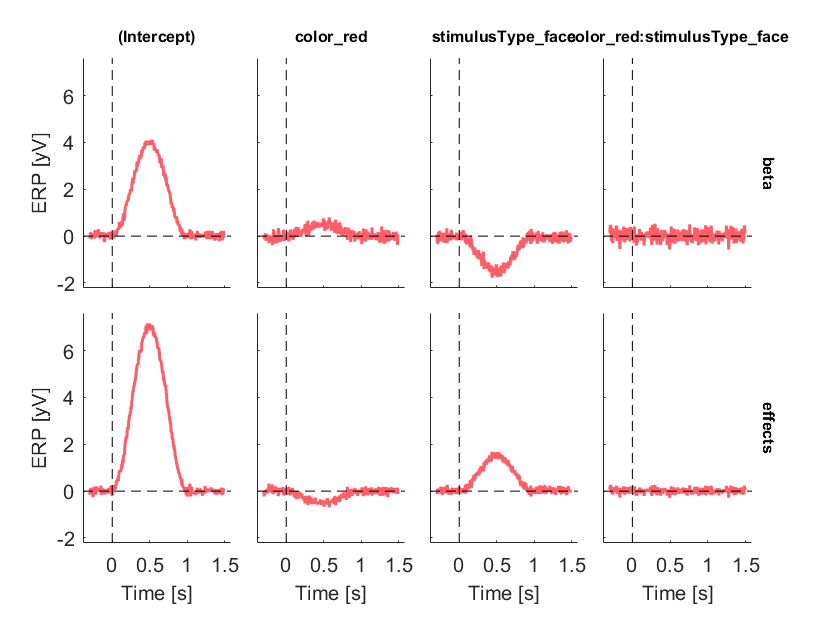As you can see, in this case two things change: The intercept is larger because with effect-coding (second row) it actually represents the average of the group-averages. Also the signs are flipped, this is just a question of the native matlab encoding. The most interesting thing about effect coding is not visible here: If an interaction is present, only effect coding directly models main effects. The columns of treatment/dummy coding would show so called simple effects. Beware of this difference. In general, if you want to recover the original ERPs, use treatment/dummy coding. If you want to interpret main effects, use effects coding!

# Recovering the modeled ERPs

Recovering the modeled ERPs is the straight forward application of a contrast (a matrix product is the same as the sum of the elementwise products).
erp_green_car = squeeze(ufresult.beta) * [1,0,0,0]'; % 1 * Intercept + 0 * red + 0 * face + 0*0 red:face
erp_red_car = squeeze(ufresult.beta) * [1,1,0,0]'; % 1 * Intercept + 1 * red + 0 * face + 1*0 red:face
figure
plot(erp_red_car,'r'), hold on
plot(erp_green_car,'g')